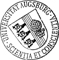## Gaussian and Non-Gaussian Stable Limit Laws in Wicksell's Corpuscle Problem

• Suppose that a homogeneous system of spherical particles (d-spheres) with independent identically distributed radii is contained in some opaque d-dimensional body, and one is interested to estimate the common radius distribution. The only information one can get is by making a cross-section of that body with an s-flat (0 <= s <= d-1) and measuring the radii of the s-spheres and heir midpoints. The analytical solution of (the hyper-stereological version of) Wicksell's corpuscle problem is used to construct an empirical radius distribution of the d-spheres. In this paper we study the asymptotic behaviour of this empirical radius distribution for s = d-1 and s = d-2 under the assumption that the intersection volume becomes unboundedly large and the point process of the midpoints of the d-spheres is Brillinger-mixing. Among others we generalize and extend some results obtained in  and  under the Poisson assumption for the special case d=3 and s=2.

Author: Lothar HeinrichGND urn:nbn:de:bvb:384-opus4-38267 https://opus.bibliothek.uni-augsburg.de/opus4/3826 Preprints des Instituts für Mathematik der Universität Augsburg (2016-04) Preprint English Universität Augsburg 2016/08/23 Wicksell's corpuscle problem; asymptotic normality; Brillinger-mixing point process; shot-noise process; characteristic function; alpha-stable distribution functions Asymptotik; Punktprozess; Schrotrauschen; Stabile Verteilung; Charakteristische Funktion Mathematisch-Naturwissenschaftlich-Technische Fakultät Mathematisch-Naturwissenschaftlich-Technische Fakultät / Institut für Mathematik Mathematisch-Naturwissenschaftlich-Technische Fakultät / Institut für Mathematik / Lehrstuhl für Stochastik und ihre Anwendungen 5 Naturwissenschaften und Mathematik / 51 Mathematik / 510 MathematikDeutsches Urheberrecht mit Print on Demand